# AnalyticBridge

A Data Science Central Community

The Monte Carlo method is an simple way to solve very difficult probabilistic problems. This text is a very simple, didactic introduction to this subject, a mixture of history, mathematics and mythology.

The method has origins in the World War II, proposed by the Polish American mathematician Stanislaw Ulam and Hungary American mathematician John Von Neumann.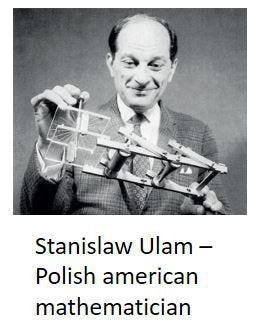It is not a coincidence these scientists were European that came to the United States. Several world class scientists did the same, escaping from the Nazis and their military domination.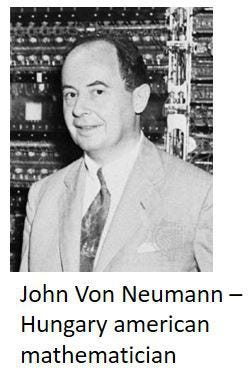These and other bright scientists were involve in the secret project of the development of the Atomic Bomb. This secret project was the Manhattan Project.

### What does the Atomic Bomb has to do with Simulation?

This is a very easy, simplified introduction, in order to give an idea of Monte Carlo method in Manhattan Project.

Imagine we have an Atom of Plutonium.

An “Atom” is a Greek word for “indivisible”. “A” = not, “tom” = tomes, divisions. Scientists believed that these unique elements of nature were the indivisible building blocks of everything in the universe, like some kind of Lego. The idea of atom has the roots on philosopher Democritus, circa 500 a.C.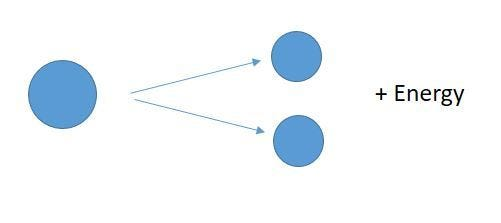In the 1930s, scientists were researching the newly discovered nuclear fission, in which an atom is broken down, divided. An atom was not more indivisible. The atom divided in two other atoms, and released a astounding amount of energy. The bomb is the energy of the atom in our hands.

The enriched Plutonium was an excellent atom for this, because it was highly unstable. And Uranium also.

Imagine a ball in the top of a mountain. Any little push will move the ball and release its potential energy into kinetic energy. That’s the meaning of instability.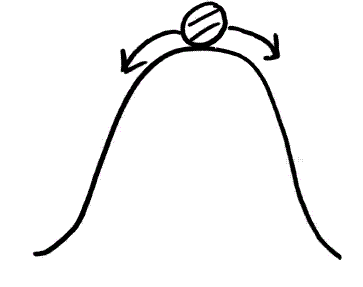But there were a lot of problems. To process the natural mineral and enrich the Plutonium was a very very painful process. It’s like to carve down an entire mountain of material, spending enormous quantities of energy, just to get a milligram of enriched Plutonium. How much of this precious Plutonium was enough? How do they could use it?

### Domino Effect

It is inoffensive to release the power of just one atom. In order to create a bomb, there is the need to create a chain reaction: one atom releases its energy, and it breaks another atom, and them another: a domino effect. It is like fire. With few gasoline, the fire will extinguish and will not produce the chain reaction to burn a forest.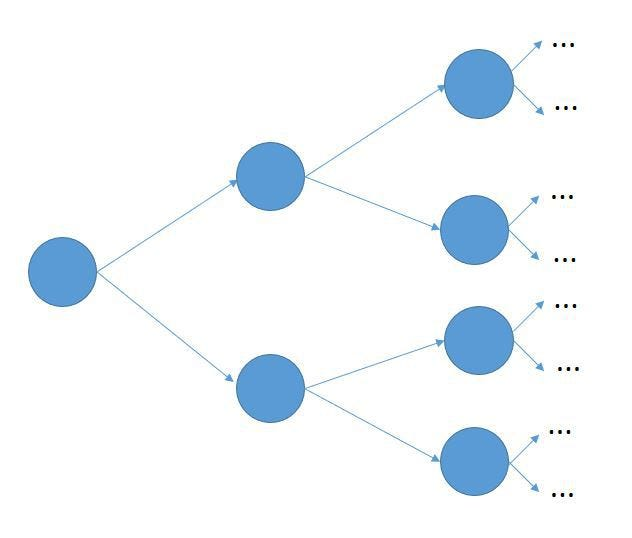The sub critical reaction is when the bomb doesn’t explode: the chain reaction doesn’t happen. It is like a domino chain that is interrupted along this way.

The super critical reaction is when the bomb explodes: there is an exponential amount of energy being released.

The mission of the scientists was to find the conditions for the critical reaction, which is the line between exploding or not.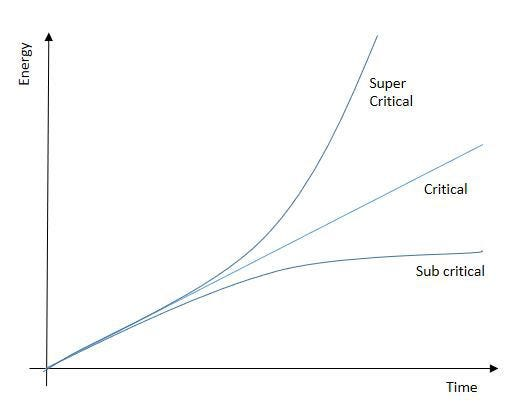### Do not explode in my hands

The knowledge of the critical condition had two main goals: they need to be assured that the bomb would explode, but also they needed to be sure that it didn’t explode in their hands.

They had to divide the amount of Plutonium in little pieces, little enough to do not explode when they didn’t want, even if an accident happens.

And they had to join the little pieces in one single great piece, with enough material to cause a chain reaction when they wanted to (in the moment of the explosion).

### How to calculate it?

How to calculate if a bomb will explode or not?

In a very simplified way, there is a model for calculation the behavior of a single atom: probability of explosion, amount of energy released for each fission of an atom, etc.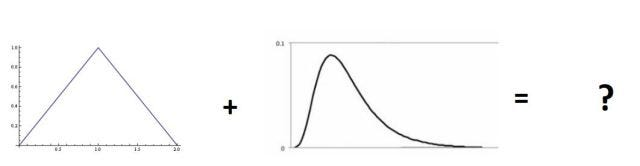If there is a model for a single atom, they needed to calculate the behavior of a group of atoms, given they are in a certain distance and density. If the first fission, will the second atom do the same? Will the third do the same?

In other words, each atom is a random variable. The compound effect in two atoms is a sum of two random variables.

To sum random variables is not an easy task. It means to solve an integral equation (not easy even for genius like Von Neumann). The sum of two random variables is manageable, but the sum of hundreds of thousands of variable, not.

Ulam and Von Neumann proposed two solutions:

1. The Monte Carlo method, with human calculators. Imagine a way do divide the scenarios in several small cases. Each case is defined by a draw on the probability distribution for each atom. Each case is calculated by a woman (men aren’t fit to this task, because them make a lot of mistakes), using paper and pencil (can you imagine this?)… and then a mathematician group together all the several cases. It was named “Monte Carlo” after the casino name, because it remembers a throw of dice. There was a room, full of women doing calculations. Note: they didn’t even know what they were calculating, since the project was secret.“Human computers”

2. The second solution was using Electronic Computers. The only problem: electronic computers didn’t exist. The solution of Von Neumann was to invent the electronic computer! Von Neumann did the conceptual architecture of the electronic computer (CPU, memory, input, output, etc), and the computer we use today still uses Von Neumann architecture. The only problem: it wasn’t completed before the war ended. Therefore, the atomic bomb is entirely due to the efforts of the human calculators.In a probabilistic world, we use random variables to represent stochastic phenomena. We choose the right random variable to represent what we want. If it is an event like the height of a group of people, we use a normal variable. If it is the arrival of clients in a queue, we usually model it by an exponential function. If we have no idea, but somehow we know the minimum and maximum, the uniform distribution is a good choice.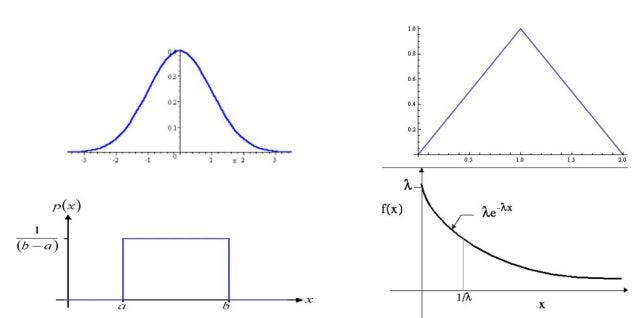A very common mistake is to use a very complicated function unnecessarily. Say, the analyst has one year of measurements, and he has learned in college a weibull distribution that fits well. Is it a good choice? No, it is not, unless he knows exactly what he is doing. He will be overfitting the model. Because we don’t want to model the past. We want to model the future. And the future will not necessarily fit in a complicated weibull. I prefer to be humble, and say “I don’t know exactly”. The way we say “I don’t know exactly” is to use a very simple random variable, a normal, an uniform.

Once we know the random variables, we can use the Monte Carlo method. It consists in throwing a dice on this random variable. Depending on the value we get from the dice, we get the value of the random variable.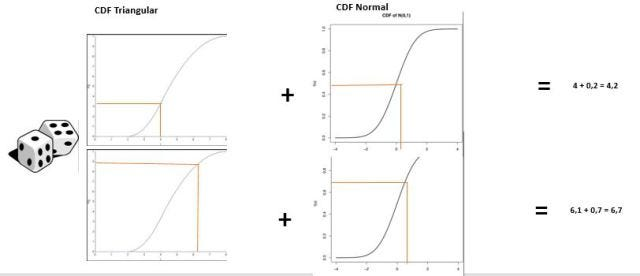In the first example, we get 4 from the first throw, and 0.2 from the second throw, resulting in 4.2.

In the second example, we get 6.1 from the first throw, and 0.7 from the second throw, resulting in 6.7.

If we do this a million times, we can estimate the distribution of probabilities of the final random variable. Each step is very very easy, easy enough to be done by a human calculator, or a electronic computer. This way, we can model a very complicated model in a simple way.

The Monte Carlo method is a fine way to find the variations of the process. In other words, the risk of the process. The risk of a supply chain to be understock or overstock. The risk of the costs of the project to be above budget. The risk of the work to exceed timeline. And so on.

Today, in a single laptop, we have the processing power equivalent to millions of humans doing calculations by hand. In a simple Excel spreadsheet, we can have very very complicated models. We have the power of computation in our hands.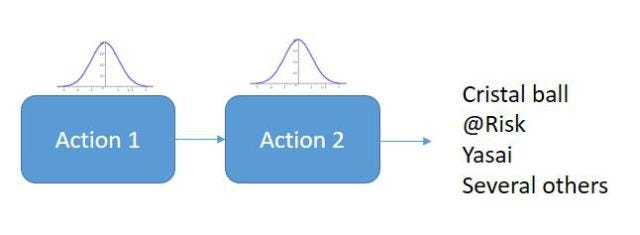Today, the bottleneck is not the computation, but the hypothesis of the model itself.
— The data also must have quality, popularly, garbage in, garbage out.
— The model must be simple enough, because of complexity — a complex model may have thousands of parameters, making it impossible to be analyzed and debugged. But it also needs to be complex enough to represent reality in a reasonable way.

No shelf software can do a good simulation for every possible situation. It is the art of modelling.

### Plutonium and Uranium

The scientists of Manhattan Project were researching two atoms: uranium and plutonium. In the end they made two bombs.

— The Hiroshima bomb was the “Little boy”, an uranium bomb.
— The Nagasaki bomb was the “Fat man”, a plutonium bomb.

Uranus is the god of the sky, in the Greek mythology. Pluto is the god of the hell. Two bombs. Sky and Hell.

Views: 13289

Tags: Carlo, John, Monte, Neumann, Stanislaw, Ulam, Von

Comment